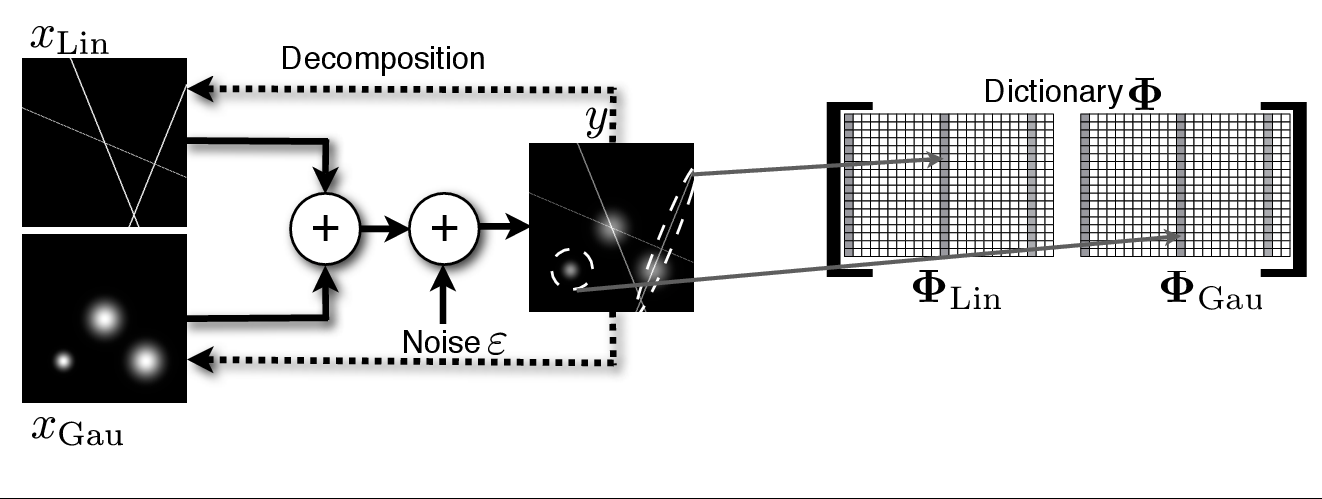# Morphological Component Analysis

One fundamental problem in signal processing involves decomposing an image or signal into superposed contributions from different sources. Morphological Component Analysis (Starck et al. 2004) addresses this class of problems using sparsity as a tool to differentiate several contributions in a signal, each one having a specific morphology.

[metaslider id=1863]

See more illustrations on the MCA Experiments page.

## The MCA Framework

Formally, the observed signal $y$ is modelled as a noisy linear mixture of distinct morphological components $\left(x_k \right)_{k=1,\cdots,K}$:

MCA assumes that a dictionary can be built by amalgamating several sub-dictionaries $\left(\Phi_1,\cdots,\Phi_K\right)$ such that, for each $k$, the representation of $x_k$ in $\Phi_k$ is sparse and not, or at least not as sparse, in other $\Phi_l, l \ne k$. In other words, the sub-dictionaries $\left(\Phi_k\right)_k$ must be mutually incoherent. Thus, the dictionary $\Phi_k$ plays a role of a discriminant between content types, preferring the component $x_k$ over the other parts.Illustration of the image decomposition problem with sparse representation.

Figure 1 gives an illustrative example. Let our image contain lines and Gaussians. Then the ridgelet transform (here $\Phi_{Lin}$) would be very effective at sparsely representing the global lines and poor for Gaussians. On the other hand, wavelets (here $\Phi_{Gau}$)  would be very good at sparsifying the Gaussians and clearly worse than ridgelets for lines.

To effectively solve the separation problem, Starck et al. (2004b) and Starck et al. (2005a) proposed the MCA algorithm which estimates each component $\left(x_k \right)_{k=1,\cdots,K}$ by solving the following optimization problem:

where $\parallel \alpha \parallel^p_p$ is sparsity-promoting (the most interesting regime is for $0 \leq p \leq 1$), and $\sigma$ is typically chosen as a constant times $\sqrt{N}\sigma_\epsilon$.